# Non Linearity! vs Parametric

Is it true that the only way to achieve above Unity is through Non Linear Effects?

### What's the Importance?

Simplicity! One could say that Parametric is the same thing as Non Linearity in Electrical Engineering terms, they mean the same thing at a base level. A Circuit or Electrical Component exhibiting Non Linear Effects will have the Value of a Component or the entire Circuit change at one point compared to the next. Parametric is the same thing. If we had a Parametric Oscillator, the Value of a Component, typically the Inductance but not always, will change from one point in time to the next. To see a visual representation, a Graph or a Non Linear Electrical Component over time would not show a straight line. A Graph or a Parametric Electrical Component over time would not show a straight line as well.

### Energy Stored in an Inductor:

The Energy Stored in the form of a Magnetic Field in an Inductor is calculated as follows: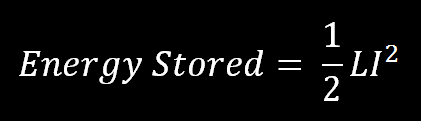The Time to charge an Inductor is shown here: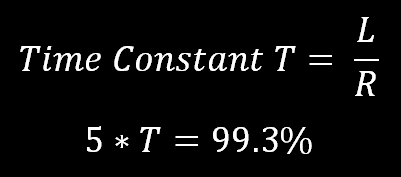An Inductor will take time to charge. Voltage is ahead of the flow of Current by 90 Degrees. An Inductor is at 99.3% of its maximum Charge Capacity (Maximum Field for the applied Voltage), in Five Time Constants. This means keeping the Inductor connected to a Current Source for longer than this time is not really going to do you any good if all you want to do is charge the inductor.

IMPORTANT: If the Coil is NOT in Resonance, We have two other factors to take into account. Inductive Capacitance, and Inductive Reactance. If the Coil is in Resonance, XC and XL are EQUAL and they cancel each other out. Well sort of, XC is considered negative and XL Positive, one will cancel the other out and this means that there is Zero Impedance in a ideal circuit.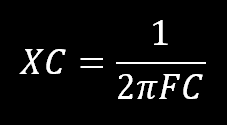Typically XC will be predominant before the frequency of Resonance and XL will be predominant after the frequency of Resonance. XL is proportional the Frequency, faster the frequency the higher the value of XL will be because XL is the opposition to Change in the Inductor due to Self-Inductance (The Self Generation of Currents flowing in the Inductor that Oppose the initial flow of current that created them).

### Parametric Inductance!

If the Series Resonant Circuit LC Circuit were at resonance, XC and XL cancel and the Impedance is Zero, then we can look at Parametric operation with not to many other factors in the equation. Resonance can be calculated with this Equation: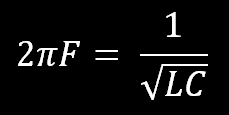Capacitive Resonance is not anywhere near as efficient as Inductive Resonance so a lower value of capacitance will mostly be more efficient with a larger inductance.

If we come back to our first Equation, we will see that if we can efficiently change the Inductance (L) during part of the resonance action then by increasing L (by inserting an Iron core into the coil for example) will increase the the Stored Energy in the Inductor. At the same time, the Resonance will change but if we remove the inductor at the right time then the resonance will stay the same. So how you may ask?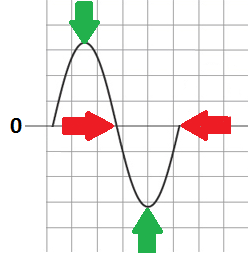From Zero to the first Green Arrow, Inductance would be lowest and there would be NO Core material. Then Instantaneously, we would introduce an Iron Core, Inductance goes up and the Stored Energy would also go up as a result. This part of the Action is where the Parametric Change has happened at the same time introducing Non Linearity into the Action. The Action then moves toward Zero again.  Instantaneously we remove the Iron Core and the Inductance drops back to its original value. Thus, the Action carries on and the device is Non Linear, or exhibits Parametric Properties, again, the same thing as we mentioned above.

So some Math: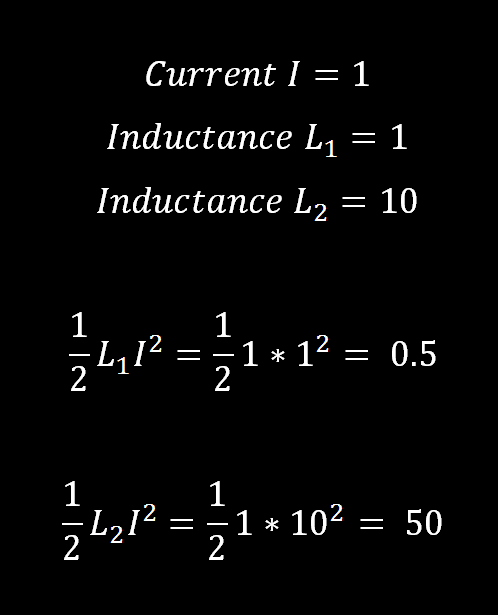This means, plugging in some simple figures, figures that are very conservative, to keep the math simple, because my math is only simple, that we can see a gain. by increasing the inductance, the math shows there should be more stored energy in the inductor. The question is, is there a way to do this efficiently enough so we can see this gain? It may be possible, if the Knee of Saturation was the working area, that we may be able to work in this area. To use a small DC  Bias, and a DC Pulse on top of this Bias to just before the point of Saturation may exhibit these sorts of things.

Inductance DECREASES as the Magnetic Field is increased and at the point of Saturation the Core is no longer able to support more flux. The Inductance moves to the value of an AIR Core (No Core) and as the Flux decreases the inductance Increases. This is a very NON Linear region. Closed Core Inductors (EG: Transformers) will hold onto residual Magnetic Field and this experiment will not work very well. A small Gap will allow the Core Material to return close to its state before you started.

I wish to point out, I am only doing research on these ideas. I have only done a few experiments to date. My aim is to try to find areas where I might see something amazing and try not to just stumble and fall.

Definition:

Ref: "http://www.thefreedictionary.com/nonlinear"

• By: hyiq.org (Chris)
• Date Created: 10/01/2014 1:10:58 PM
• Date Modified: 10/01/2014 1:32:35 PM